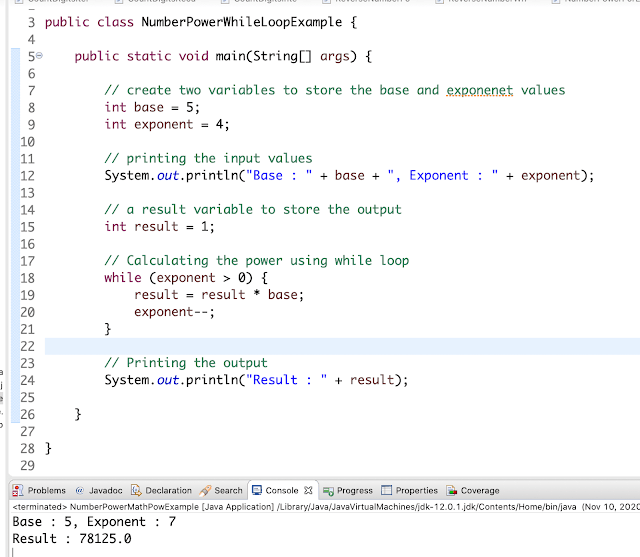## \$show=/label

A quick programming guide to calculate the power of a number without using Math.pow() method. Example, find power of number using for and while loop.

## 1. Overview

In this tutorial, you are going to learn how to find the power of a number without using Math.pow() method.

But, the power of a number can be solved with the help of for loop and while loops.

Example:

Input: Base = 4, Exponent : 3
Output: 63

Finding the power is to multiply the base with exponent value that means here base is 4 and exponent is 3.
So, power calculation is 4 * 4 * 4 = 64.

## 2. Example 1 - Power Of A Number Using For Loop

In the below program, first created two integer type variables base and exponent.

Next, run through the for loop for exponent times and multiply the base value those many times.
```public class NumberPowerForLoopExample {

public static void main(String[] args) {

// create two variables to store the base and exponenet values
int base = 4;
int exponent = 3;

// printing the input values
System.out.println("Base : " + base + ", Exponent : " + exponent);

// a result variable to store the output
int result = 1;

// Calculating the power using for loop
for (int i = 1; i <= exponent; i++) {
result = result * base;
}

// Printing the output
System.out.println("Result : " + result);

}

}
```
Output:
```Base : 4, Exponent : 3
Result : 64
```

## 3. Example 2 - Power Of A Number Using While Loop

Next, run the same logic using while loop until the exponent is 0.

Look at the below example and output.
```public class NumberPowerWhileLoopExample {

public static void main(String[] args) {

// create two variables to store the base and exponenet values
int base = 5;
int exponent = 4;

// printing the input values
System.out.println("Base : " + base + ", Exponent : " + exponent);

// a result variable to store the output
int result = 1;

// Calculating the power using while loop
while (exponent > 0) {
result = result * base;
exponent--;
}

// Printing the output
System.out.println("Result : " + result);

}

}
```
Output:
```Base : 5, Exponent : 4
Result : 625
```

## 4. Example 3 - Power Of A Number Using Math.pow() Method

In the last approach, you can use the java Math API builtin method pow(base, exponent) which does the calculation of power of a given number for the given exponent.

This can be done using recursive approach
```package com.javaprogramto.programs.numbers;

public class NumberPowerMathPowExample {

public static void main(String[] args) {

// create two variables to store the base and exponenet values
int base = 5;
int exponent = 7;

// printing the input values
System.out.println("Base : " + base + ", Exponent : " + exponent);

// a result variable to store the output
double result = 1;

// Calculating the power using Math.pow() method
result = Math.pow(base, exponent);

// Printing the output
System.out.println("Result : " + result);
}
}
```

Output:
```Base : 5, Exponent : 7
Result : 78125.0
```

## 5. Conclusion

In this article, you've seen the three ways to find the power of a number.

All examples are over GitHub.BLOGGER

Author: Venkatesh - I love to learn and share the technical stuff.
Name

accumulo,1,ActiveMQ,2,Adsense,1,API,37,ArrayList,18,Arrays,24,Bean Creation,3,Bean Scopes,1,BiConsumer,1,Blogger Tips,1,Books,1,C Programming,1,Collection,8,Collections,37,Collector,1,Command Line,1,Comparator,1,Compile Errors,1,Configurations,7,Constants,1,Control Statements,8,Conversions,6,Core Java,149,Corona India,1,Create,2,CSS,1,Date,3,Date Time API,38,Dictionary,1,Difference,2,Download,1,Eclipse,3,Efficiently,1,Error,1,Errors,1,Exceptions,8,Fast,1,Files,17,Float,1,Font,1,Form,1,Freshers,1,Function,3,Functional Interface,2,Garbage Collector,1,Generics,4,Git,9,Grant,1,Grep,1,HashMap,2,HomeBrew,2,HTML,2,HttpClient,2,Immutable,1,Installation,1,Interview Questions,6,Iterate,2,Jackson API,3,Java,32,Java 10,1,Java 11,6,Java 12,5,Java 13,2,Java 14,2,Java 8,128,Java 8 Difference,2,Java 8 Stream Conversions,4,java 8 Stream Examples,12,Java 9,1,Java Conversions,14,Java Design Patterns,1,Java Files,1,Java Program,3,Java Programs,114,Java Spark,1,java.lang,4,java.util. function,1,JavaScript,1,jQuery,1,Kotlin,11,Kotlin Conversions,6,Kotlin Programs,10,Lambda,2,lang,29,Leap Year,1,live updates,1,LocalDate,1,Logging,1,Mac OS,3,Math,1,Matrix,6,Maven,1,Method References,1,Mockito,1,MongoDB,3,New Features,1,Operations,1,Optional,6,Oracle,5,Oracle 18C,1,Partition,1,Patterns,1,Programs,1,Property,1,Python,2,Quarkus,1,Read,1,Real Time,1,Recursion,2,Remove,2,Rest API,1,Schedules,1,Serialization,1,Servlet,2,Sort,1,Sorting Techniques,8,Spring,2,Spring Boot,23,Spring Email,1,Spring MVC,1,Streams,31,String,61,String Programs,28,String Revese,1,StringBuilder,1,Swing,1,System,1,Tags,1,Threads,11,Tomcat,1,Tomcat 8,1,Troubleshoot,26,Unix,3,Updates,3,util,5,While Loop,1,
ltr
item
JavaProgramTo.com: Java Program to Calculate the Power of a Number With Examples
Java Program to Calculate the Power of a Number With Examples
A quick programming guide to calculate the power of a number without using Math.pow() method. Example, find power of number using for and while loop.